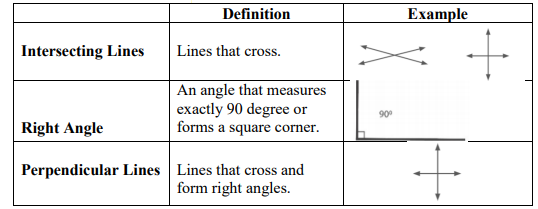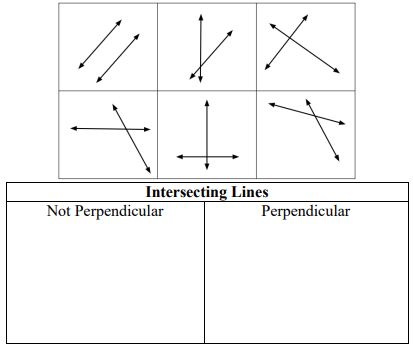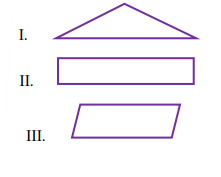# Standard #: MA.3.GR.1.1

This document was generated on CPALMS - www.cpalms.org

Describe and draw points, lines, line segments, rays, intersecting lines, perpendicular lines and parallel lines. Identify these in two-dimensional figures.

### Clarifications

Clarification 1: Instruction includes mathematical and real-world context for identifying points, lines, line segments, rays, intersecting lines, perpendicular lines and parallel lines.

Clarification 2: When working with perpendicular lines, right angles can be called square angles or square corners.

### General Information

Subject Area: Mathematics (B.E.S.T.)
Strand: Geometric Reasoning
Status: State Board Approved

### Terms from the K-12 Glossary

• Line
• Parallelogram
• Rectangle
• Square
• Triangle

### Vertical Alignment

Previous Benchmarks

Next Benchmarks

### Purpose and Instructional Strategies

The purpose of this benchmark is to build important vocabulary that allows students to describe and draw points, lines, line segments, rays, intersecting lines, perpendicular lines and parallel lines, and to identify examples in two-dimensional figures represented in mathematical and real- world contexts. In Grade 2, students were expected to identify and draw two-dimensional figures based on their defining attributes. In Grade 3, students can describe and draw geometric figures using more formal vocabulary developed in this benchmark. Therefore, instruction of this benchmark relies heavily on vocabulary development for students to internalize definitions and make connections between the concepts. In Grade 4, students will explore and draw angles beyond square angles.
• In mathematical contexts, students find evidence of points, lines, line segments, rays, intersecting lines, perpendicular lines and parallel lines in models of two-dimensional figures (e.g., quadrilaterals, triangles). In real-world contexts, students identify evidence of these geometric attributes in real-life images (e.g., aerial views of city maps, photos of objects) (MTR.4.1, MTR.7.1).
• This vocabulary development will be necessary as students identify and draw quadrilaterals based on their defining attributes (MA.3.GR.1.2). It will also be beneficial in other areas as students begin to read, draw and understand graphs and diagrams.
• Instruction should also consider activities that encourage student discussions rich in mathematical reasoning opportunities. Mathematical discussions and reasoning activities give students the practice necessary to use the vocabulary and internalize it in meaningful ways. An example of a mathematical reasoning activity that builds vocabulary understanding is in the instructional task below (MTR.4.1).
• Images of figures used in instruction should not include hatch marks.
• Because formal instruction of angle measurements does not begin until Grade 4, students can refer to right angles in perpendicular lines as “square angles” or “square corners.”

### Common Misconceptions or Errors

• Students can confuse some pairs of intersecting lines as perpendicular. Encourage students to justify their thinking whenever they reason about geometric concepts. For example, students can use the corners of a standard sheet of paper as a comparison to determine whether a pair of intersecting lines is perpendicular.
• Students may struggle to identify examples of points, lines, line segments, rays, intersecting lines, perpendicular lines and parallel lines in real-world examples. Classroom instruction should include many examples for students to explore.

### Strategies to Support Tiered Instruction

• Instruction provides opportunities to justify thinking when reasoning about geometric concepts.
• For example, the teacher demonstrates how to use the corners of a standard sheet of paper as a comparison to determine whether a pair of intersecting lines is perpendicular.
• Instruction includes the use of key vocabulary, referencing definitions for terms such as intersecting lines, right angle, and perpendicular lines. The teacher draws examples of intersecting lines that are both perpendicular and not perpendicular and has students explain which they are and justify their reasoning.
• For example, teachers provide key vocabulary as shown below for students to refer to. The teacher will then draw sets of lines, some that do not intersect, some that intersect but do not create right angles, and other sets that do create right angles. Students determine which pairs of intersecting lines can be classified as perpendicular and explain why.• The teacher provides a tool such as a square tile or the corner of a piece of paper to identify intersecting lines that create right angles and classify those as perpendicular lines and those that do not form right angles as intersecting but not perpendicular. Students use the tool to draw some of their own intersecting lines that would be examples of both.
• For example, the teacher may provide students with a graphic organizer like the one shown below and a set of cards with pairs of lines (examples shown below). The students use the tool to sort the cards into perpendicular and not perpendicular and draw at least one pair of their own lines for each category.• Instruction includes real-world examples of points, lines, line segments, rays, intersecting lines, perpendicular lines, and parallel lines. The teacher provides images of real-world examples that include geometric figures. Students identify the geometric figure in the example.
• For example, the teacher provides an image of railroad tracks to represent parallel lines, a speed sign to represent perpendicular lines, a balance beam to represent a line segment, and other common images.
• Instruction includes real-world examples of points, lines, line segments, rays, intersecting lines, perpendicular lines, and parallel lines. The teacher points out items in the classroom that are examples of the geometric terms listed above and has students identify which term it is an example of.
• For example, if the teacher points out a poster with the number one or the letter l on it, students will say it represents a line segment. If the teacher points out the window, students will say the top and bottom of the window shows parallel lines, while the corners of the window show perpendicular lines.
• For example, students to find their own examples within in the classroom and explain which geometric term they notice in the figure.

Are intersecting lines always, sometimes or never parallel? Show your thinking.

Are intersecting lines always, sometimes or never perpendicular? Show your thinking.

Draw a geometric figure with parallel and perpendicular sides.

### Instructional Items

Instructional Item 1

Which of the following figures show perpendicular lines?• a. I only
• b. II only
• c. II and III
• d. I, II and III

*The strategies, tasks and items included in the B1G-M are examples and should not be considered comprehensive.

#### Related Courses

 Course Number1111 Course Title222 5012050: Grade Three Mathematics (Specifically in versions: 2014 - 2015, 2015 - 2022, 2022 and beyond (current)) 7712040: Access Mathematics Grade 3 (Specifically in versions: 2014 - 2015, 2015 - 2018, 2018 - 2022, 2022 and beyond (current)) 5012055: Grade 3 Accelerated Mathematics (Specifically in versions: 2019 - 2022, 2022 and beyond (current)) 5012015: Foundational Skills in Mathematics 3-5 (Specifically in versions: 2019 - 2022, 2022 and beyond (current))

#### Related Access Points

 Access Point Number Access Point Title MA.3.GR.1.AP.1 Identify points, lines, line segments, perpendicular lines and parallel lines. Identify these in two-dimensional figures.

#### Formative Assessments

 Name Description Parallel and Perpendicular Sides Students are asked to identify parallel and perpendicular sides and explain how they know. Locating Points, Lines, and Rays Students locate points, lines, line segments, and rays in a given diagram. Lines, Rays, and Line Segments Students are asked to draw parallel lines, perpendicular lines, a point, and a line segment. Students also explain how a line segment is different from a ray or line.

#### Original Student Tutorials

 Name Description Shape Surfing: Finding Parallel and Perpendicular Lines Help a surfing crab learn how to find parallel and perpendicular sides in a variety of polygons as you complete this interactive tutorial! The Geometry Super Heroes Save Mathopolis Identify parallel lines and line segments, as well as perpendicular lines and line segments in two-dimensional figures by joining Parallel Man and Perpendicular Man as they help Mayor Mathematics save Mathopolis in this interactive tutorial.

#### Teaching Idea

 Name Description An Introduction To Quadrilaterals This lesson is designed to introduce students to quadrilaterals and the terms and properties associated with quadrilaterals. This lesson provides links to discussions and activities related to quadrilaterals as well as suggested ways to integrate them into the lesson. Finally, the lesson provides links to follow-up lessons designed for use in succession with the current one.

#### Tutorials

 Name Description Parallel and Perpendicular Lines Introduced This Khan Academy tutorial video defines and illustrates parallel and perpendicular lines. Lines, Line segments and Rays This video discusses the differences between lines, line segments and rays.

#### Original Student Tutorials

 Name Description Shape Surfing: Finding Parallel and Perpendicular Lines: Help a surfing crab learn how to find parallel and perpendicular sides in a variety of polygons as you complete this interactive tutorial! The Geometry Super Heroes Save Mathopolis: Identify parallel lines and line segments, as well as perpendicular lines and line segments in two-dimensional figures by joining Parallel Man and Perpendicular Man as they help Mayor Mathematics save Mathopolis in this interactive tutorial.

#### Tutorials

 Name Description Parallel and Perpendicular Lines Introduced: This Khan Academy tutorial video defines and illustrates parallel and perpendicular lines. Lines, Line segments and Rays: This video discusses the differences between lines, line segments and rays.

Printed On:10/2/2023 2:06:28 AM
Print Page | Close this window